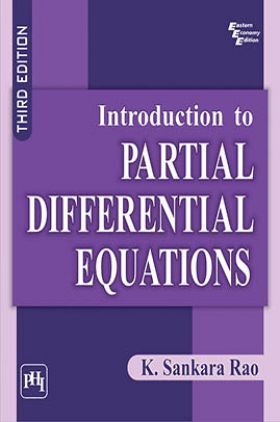# Introduction To Partial Differential Equations

( 52 )
Sold ( 81 times )
26718 Views

This product is currently not available.

Save extra with 1 Offers

Get ₹ 50

Instant Cashback on the purchase of ₹ 400 or above

Product Specifications

 Publisher PHI Learning All Engineering Mathematics books by PHI Learning ISBN 9788120342224 Author: RAO, K. SANKARA Number of Pages 508 Edition Third Edition Available Available in all digital devicesAbout The Book Introduction To Partial Differential Equations

Book Summary:

This comprehensive and well-organized book, now in its Third Edition, continues to provide the students with the fundamental concepts, the underlying principles, various well-known mathematical techniques and methods such as Laplace and Fourier transform techniques, the variable separable method, and Greens function method to solve partial differential equations.

The text is supported by a number of worked-out examples and miscellaneous examples to enable the students to assimilate the fundamental concepts and the techniques for solving partial differential equations with various initial and boundary conditions. Besides, chapter-end exercises are also provided with hints to reinforce the students skill.

It is designed primarily to serve as a textbook for senior undergraduate and postgraduate students pursuing courses in applied mathematics, physics and engineering. Students appearing in various competitive examinations like NET, GATE, and the professionals working in scientific R&D organizations would also find this book both stimulating and highly useful.

What is new to this edition ?

Adds new sections on linear partial differential equations with constant coefficients and non-linear model equations.

Offers additional worked-out examples and exercises to illustrate the concepts discussed.

Preface
Preface to the Second Edition
0. PARTIAL DIFFERENTIAL EQUATIONS OF FIRST ORDER
1. FUNDAMENTAL CONCEPTS
2. ELLIPTIC DIFFERENTIAL EQUATIONS
3. PARABOLIC DIFFERENTIAL EQUATIONS
4. HYPERBOLIC DIFFERENTIAL EQUATIONS
5. GREENS FUNCTION
6. LAPLACE TRANSFORM METHODS
7. FOURIER TRANSFORM METHODS
Bibliography